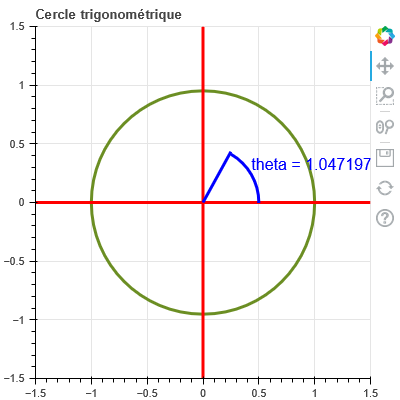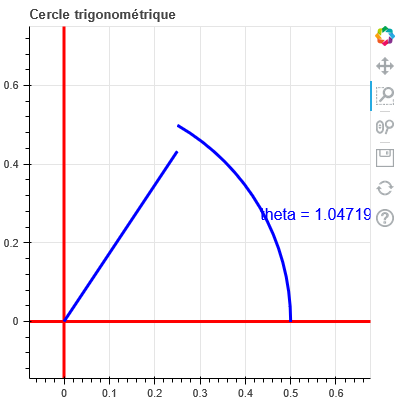# About arc and line

I have got this page Cercle trigonométrique
There is in blue an arc and a line :

``````    source_1 = ColumnDataSource(dict(x=x, y=y, r=r, theta=[theta]))
source_2 = ColumnDataSource(dict(x=[0,r*np.cos(theta)], y=[0, r*np.sin(theta)]))
source_3 = ColumnDataSource(dict(x=[r*np.cos(theta/2)], y=[r*np.sin(theta/2)], texte=['theta = '+str(theta)]))
secteur_arc = Arc(x="x", y="y", radius="r",
start_angle=0.0, end_angle="theta",
line_color="blue",
line_width=3,
direction ='anticlock'
)
secteur_line = Line(x="x", y="y",
line_color="blue",
line_width=3,
)
secteur_text = Text(x="x", y="y",
text_color="blue",
text="texte"
)

``````

Resul isnow if you use box zoomWhat’s wrong in my code?

@LaurentBerger You need to pass `match_aspect=True` to `figure` to explicitly ask Bokeh to maintain a 1-1 correspondence between data and pixel aspect ratios. Without it, the space taken up by axes, toolbars, etc, can cause them to diverge.

Do you mean here?

@LaurentBerger Yes, but please refer to the docs for `match_aspect` for additional requirements. Specifically, you will not be able to use manual `x_range` and `y_range` with `match_aspect`, only auto data-ranges. (Bokeh has to have control to tweak the range extents in order to actually make the aspect ratios match, and also to keep them that way if there is panning, zooming, etc).

If you can’t give up manual range extents, then your only option will be to very carefully and manually control an overall fixed canvas size, all the `min_border` settings together with expected space that axis ticks up, in order to make the central plot area pixel aspect ratio match that data aspect ratio. But that will be much more fragile and tedious.

Thanks I changed code (no _range)
Wheel zoom seems better than box zoom
Box zoom is really weird.

By default box zoom is unrestricted. There is a separate `match_aspect` property of box zoom tools to dictate whether the tool should only conform to the current plot.

This topic was automatically closed 90 days after the last reply. New replies are no longer allowed.